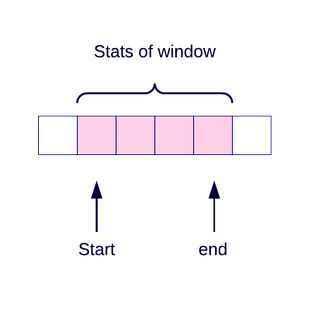Given an unsorted array A of size N of non-negative integers, find a continuous sub-array which adds to a given number S.

Input:

The first line of input contains an integer T denoting the number of test cases. Then T test cases follow. Each test case consists of two lines. The first line of each test case is N and S, where N is the size of array and S is the sum. The second line of each test case contains N space-separated integers denoting the array elements.

Output:

For each test case, in a new line, print the starting and ending positions(1 indexing) of first such occurring subarray from the left if sum equals to subarray, else print -1.

In this problem, we need to find in an array of the integers of any sub-array adds to the given sum.We will be using the Sliding Window method to solve this problem.

Sliding Window method states that we take a fixed size sub-array called a window and slide it over the given array. During the process, if at any instant the sum of the integers covered by the window equals the given sum, we return otherwise we keep on sliding the window till we reach the end of the given array.

We have used this technique in solving the given problem. What we do is, we first start adding the integers from the index = 0 of the given array till we get the sum of integers greater than equal to the given sum.

Once this condition is met, if the calculated sum == given sum, then return the indexes of the sub-array else, delete the elements from the beginning of the window, till the sum of the integers of the window is less than the given sum and after this start adding the elements from the end side of the array. This way our window slides along the given array and tries to find the given sum.

Below given code is in C programming language.

```#include<stdio.h>
void subArrayIndexes(int *arr, int sum, int n){
int window_size=arr,start=0,i;
for(i = 1 ; i < n ; i++){
while(window_size > sum && start < i-1){
window_size = window_size - arr[start]; start++;
}

if(window_size == sum){
printf("%d %d\n", start+1, i);
return;
}

window_size = window_size + arr[i];
}
printf("-1\n");
}
int main(){
int t,n,a,b,sum,arr;
scanf("%d", &t);
for(a = 0 ; a < t ; a++){
scanf("%d %d", &n, &sum);
for(b = 0 ; b < n ; b++){
scanf("%d", &arr[b]);
}
subArrayIndexes(arr,sum,n);
}
return 0;
}
```